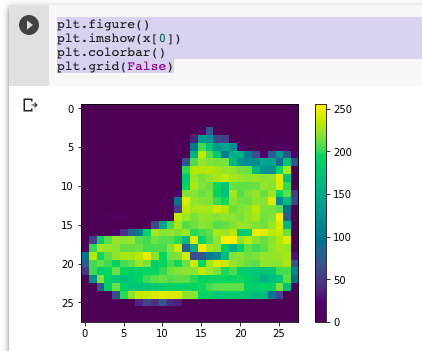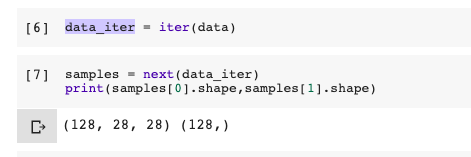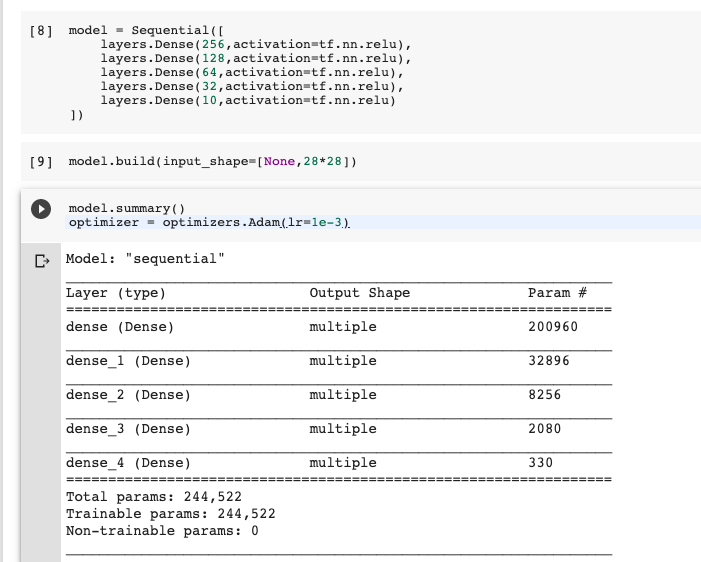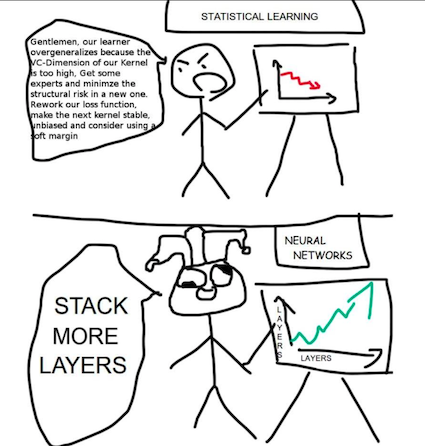#DAY 9
0

## [Day-9] Deep Neural Network (Lab: Fashion-MNIST)

Fashion MNIST

Fashion MNIST是一個涵蓋10個種類的服飾正面灰階圖片 (28*28)，主要為Zalando所release的dataset，很適合大家一開始用來嘗試各式各樣的model。而TF.keras.dataset的api已經整理很乾淨。因此，若使用的都可以直接call api，就可以下載dataset到local做使用。``````(x,y),(x_test,y_test) = datasets.fashion_mnist.load_data()
print(x.shape,y.shape)
#Output: x -> (60000, 28, 28)  y -> (60000,)
``````

``````plt.figure()
plt.imshow(x)
plt.colorbar()
plt.grid(False)
````````````data = tf.data.Dataset.from_tensor_slices((x,y))
``````

``````def feature_scale(x,y):
x = tf.cast(x,dtype=tf.float32)/255.
y = tf.cast(y,dtype=tf.int32)
return x,y

data = data.map(feature_scale).shuffle(10000).batch(128)
``````

``````data_iter = iter(data)
samples = next(data_iter)
print(samples.shape,samples.shape)
````````````model = Sequential([
layers.Dense(256,activation=tf.nn.relu),
layers.Dense(128,activation=tf.nn.relu),
layers.Dense(64,activation=tf.nn.relu),
layers.Dense(32,activation=tf.nn.relu),
layers.Dense(10,activation=tf.nn.relu)
])

model.build(input_shape=[None,28*28])

model.summary()
````````````with tf.GradientTape() as tape:
logits = model(x)
y_one_hot = tf.one_hot(y,depth=10)
loss = tf.losses.categorical_crossentropy(y_one_hot,logits,from_logits=True)
loss = tf.reduce_mean(loss)
``````

``````  x = tf.reshape(x,[-1,28*28])

gd = model(x)
prob = tf.nn.softmax(gd,axis=1)
pred = tf.argmax(prob,axis=1)

pred = tf.cast(pred,dtype=tf.int32)
correct = tf.equal(pred,y)

result = tf.reduce_sum(tf.cast(correct,dtype=tf.int32))

total_loss += int(result)
``````

### 小結：

#### 一天一梗圖：source

#### Reference

TF_data api

TF_image binary classification

Fashion_MNIST

DNN_Fashion_MNIST_Colab